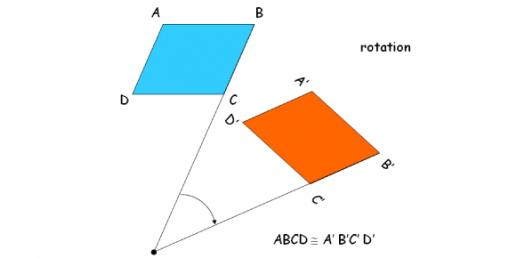# 7th Grade Geometry Trivia Quiz

10 Questions | Attempts: 536
ShareSettingsAs a part of mathematics, we all have studied geometry, haven't we? That's why we have come up with the 7th grade geometry trivia quiz for you. Geometry is, with arithmetic, known as one of the oldest branches of mathematics. It deals with properties of space like the distance, shape, size, as well as relative position of figures. These are some trivia questions that aren't going to be very difficult but fun and useful to answer. So, let's give them a try.

• 1.
Identify the coordinates of N’ if N(2,-1) is reflected through the x-axis
• A.

(2, 1)

• B.

(-2, -1)

• C.

(-2, 1)

• D.

(1, 2)

• 2.
After a reflection, the image of X(3,4) is X’(-3,4).  Therefore, the line of reflection is
• A.

The x-axis

• B.

The y-axis

• C.

Over x=1

• D.

Over y=1

• 3.
Another word for 'flip' is
• A.

Rotation

• B.

Reflection

• C.

Translation

• D.

None of these

• 4.
Radius of a _________ is the distance from the center of the circle to any point on it's circumference.
• A.

Circle

• B.

Triangle

• C.

Pentagon

• D.

Hexagon

• 5.
A triangle has three sides with the total _______ angle.
• A.

20 degree

• B.

45 degree

• C.

90 degree

• D.

180 degree

• 6.
A hexagon has ________ sides.
• A.

4

• B.

5

• C.

6

• D.

7

• 7.
A pentagon has ________ sides.
• A.

4

• B.

5

• C.

6

• D.

7

• 8.
A nonagon has ______ sides
• A.

3

• B.

7

• C.

8

• D.

9

• 9.
Another word for 'turn' is
• A.

Rotation

• B.

Reflection

• C.

Translation

• D.

None of these

• 10.
Another word for 'slide' is
• A.

Rotation

• B.

Reflection

• C.

Translation

• D.

None of these

## Related Topics

×

Wait!
Here's an interesting quiz for you.